# Calibrations

The guide on calibration gives an introduction to calibration metrics and how to retrieve them from the Google Quantum Computing Service (QCS). In this tutorial, we show how to visualize these calibration metrics via single qubit heatmaps, two qubit interaction heatmaps and integrated histograms.

Disclaimer: The calibration metrics of the quantum processor shown in the tutorial do not correspond to actual production metrics.

## Authenticate and install Cirq

For details of authentication and installation, please see Get started with Quantum Computing Service.

1. Enter the Cloud project ID you'd like to use in the `YOUR_PROJECT_ID` field.
2. Then run the cell below (and go through the auth flow for access to the project id you entered).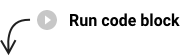```Notebook is not executed with Colab, assuming Application Default Credentials are setup.
```

### View available calibration metrics

```single_qubit_p00_error
single_qubit_p11_error
parallel_p00_error
parallel_p11_error
single_qubit_rb_average_error_per_gate
single_qubit_rb_incoherent_error_per_gate
single_qubit_rb_pauli_error_per_gate
single_qubit_idle_t1_micros
two_qubit_sycamore_gate_xeb_average_error_per_cycle
two_qubit_sycamore_gate_xeb_pauli_error_per_cycle
two_qubit_sycamore_gate_xeb_incoherent_error_per_cycle
two_qubit_sqrt_iswap_gate_xeb_average_error_per_cycle
two_qubit_sqrt_iswap_gate_xeb_pauli_error_per_cycle
two_qubit_sqrt_iswap_gate_xeb_incoherent_error_per_cycle
two_qubit_parallel_sycamore_gate_xeb_average_error_per_cycle
two_qubit_parallel_sycamore_gate_xeb_pauli_error_per_cycle
two_qubit_parallel_sycamore_gate_xeb_incoherent_error_per_cycle
two_qubit_parallel_sqrt_iswap_gate_xeb_average_error_per_cycle
two_qubit_parallel_sqrt_iswap_gate_xeb_pauli_error_per_cycle
two_qubit_parallel_sqrt_iswap_gate_xeb_incoherent_error_per_cycle
```

# Metrics

For a complete description of all the available calibration metrics, please see Calibration metrics

## Single Qubit Isolated Benchmarking

Single qubit gate error is estimated using randomized benchmarking. This error is calculated for one qubit at a time while all other qubits on the device are idle (isolated).

### Average Error

Disclaimer: The calibration metrics shown here do not correspond to actual production metrics.

``````fig, _ = cals.plot('single_qubit_rb_average_error_per_gate', fig=plt.figure(figsize=(20, 10)))
``````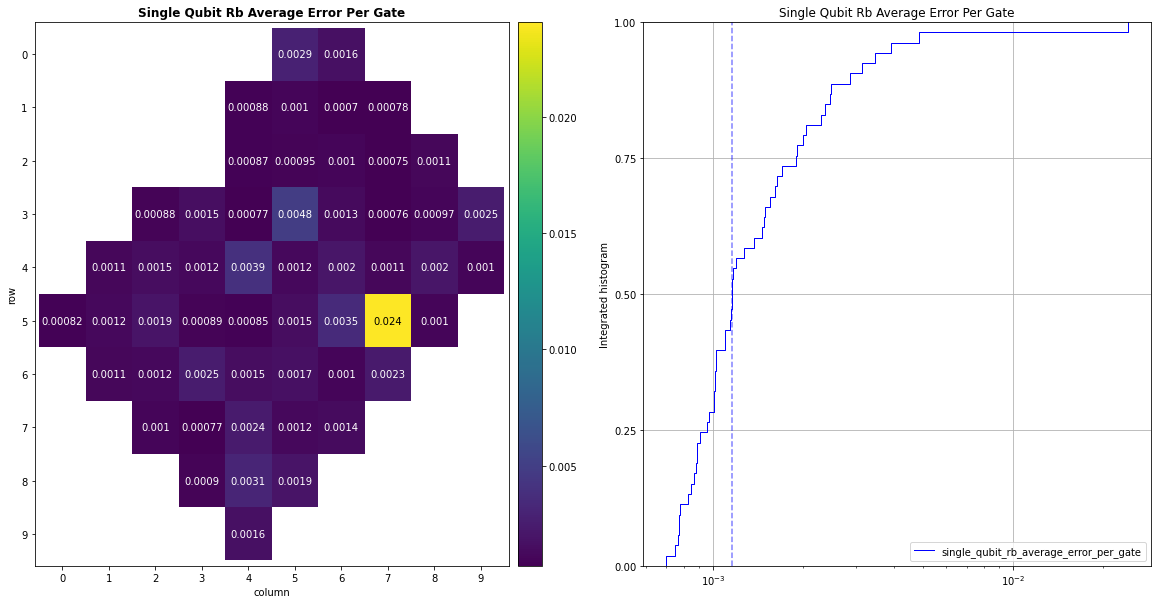### Incoherent Error

Disclaimer: The calibration metrics shown here do not correspond to actual production metrics.

``````fig, _ = cals.plot('single_qubit_rb_incoherent_error_per_gate', fig=plt.figure(figsize=(20, 10)))
``````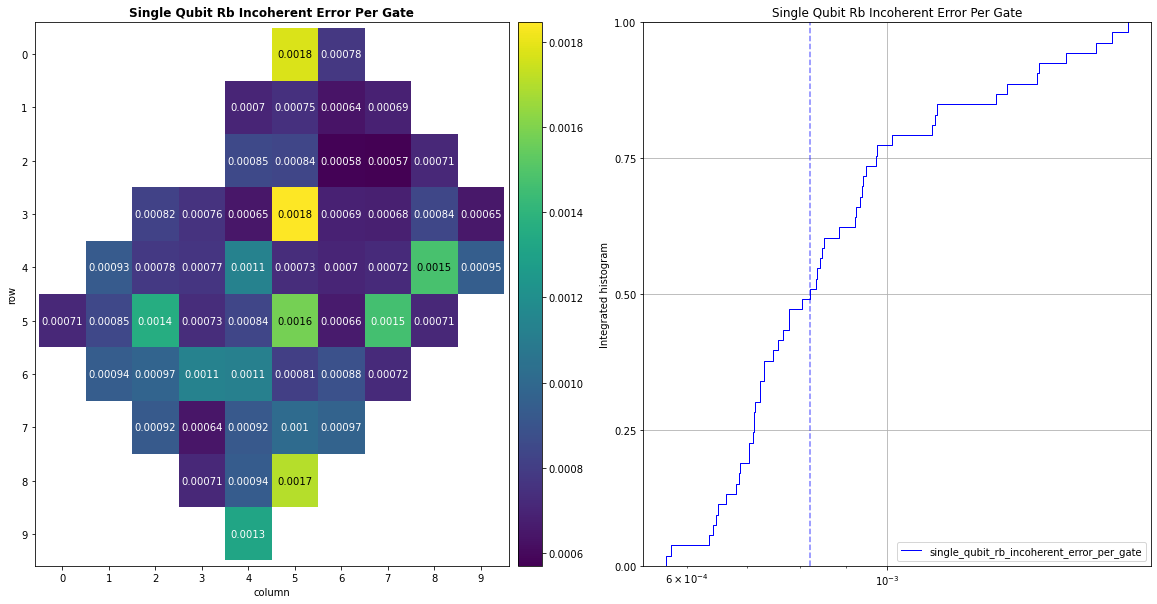### Pauli Error

Disclaimer: The calibration metrics shown here do not correspond to actual production metrics.

``````fig, _ = cals.plot('single_qubit_rb_pauli_error_per_gate', fig=plt.figure(figsize=(20, 10)))
``````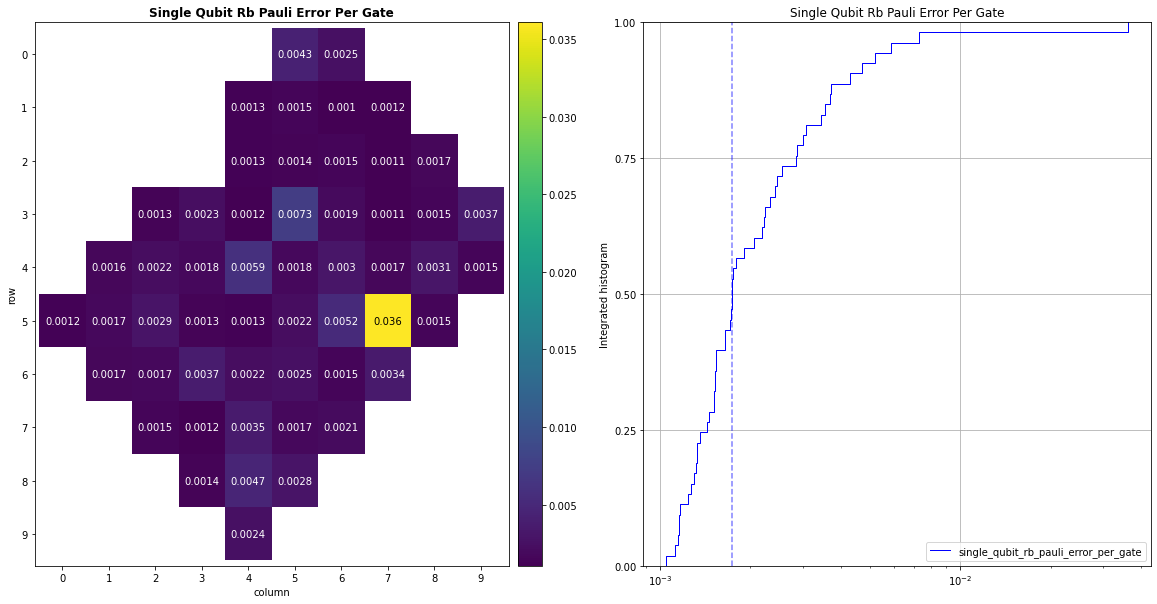### Comparison Histograms

Disclaimer: The calibration metrics shown here do not correspond to actual production metrics.

``````keys = ['single_qubit_rb_pauli_error_per_gate', 'single_qubit_rb_average_error_per_gate', 'single_qubit_rb_incoherent_error_per_gate']
fig, ax = plt.subplots(figsize=(16, 8))
cals.plot_histograms(keys, ax)
ax.set_title("Single Qubit Error Comparison Histograms")
``````
```Text(0.5, 1.0, 'Single Qubit Error Comparison Histograms')
```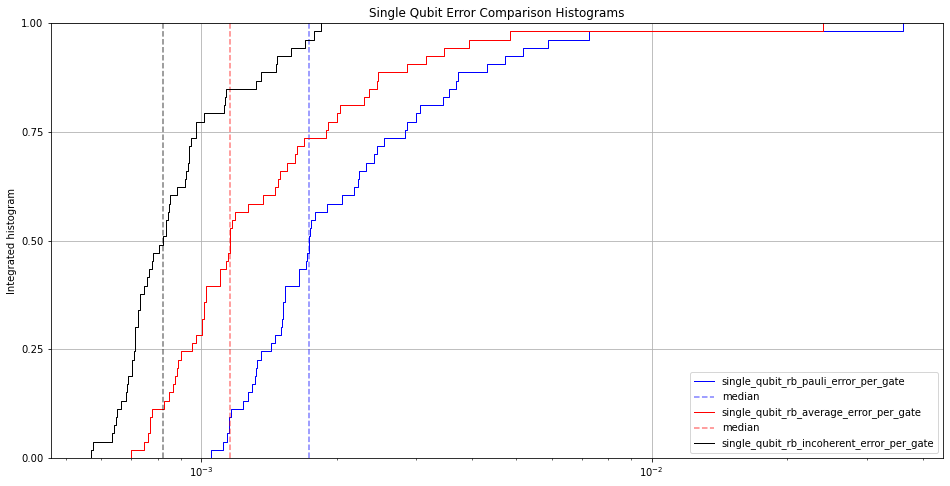The isolated single qubit error is when the readout is measured in isolation (only one qubit is measured at a time).

### Zero State Errors

Disclaimer: The calibration metrics shown here do not correspond to actual production metrics.

``````fig, _ = cals.plot('single_qubit_p00_error', fig=plt.figure(figsize=(20, 10)))
``````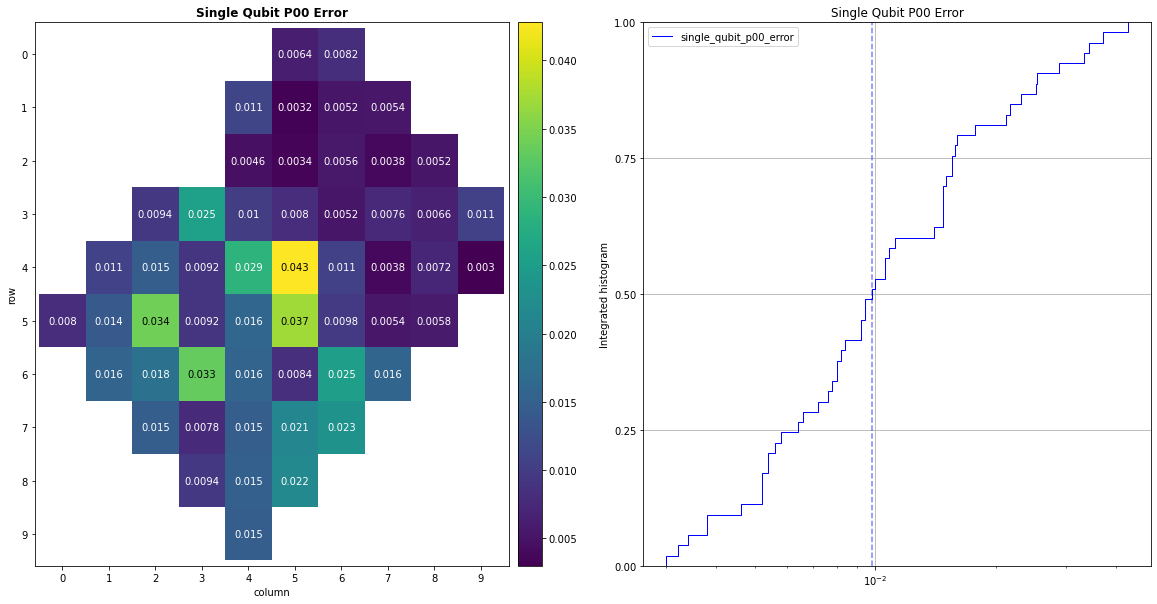### One State Errors

Disclaimer: The calibration metrics shown here do not correspond to actual production metrics.

``````fig, _ = cals.plot('single_qubit_p11_error', fig=plt.figure(figsize=(20, 10)))
``````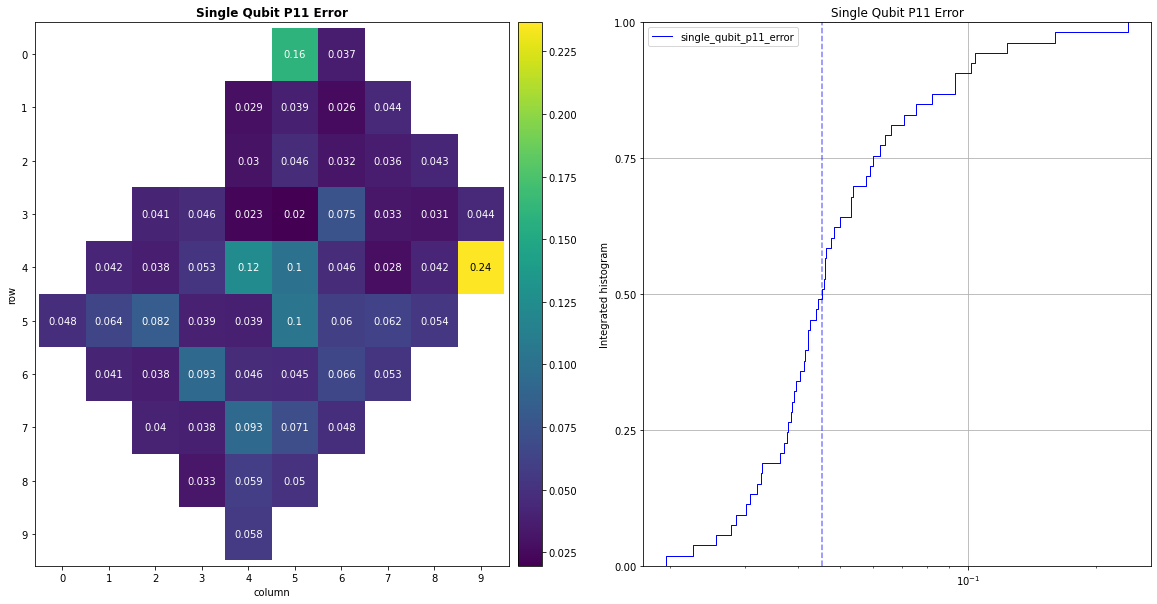Disclaimer: The calibration metrics shown here do not correspond to actual production metrics.

``````fig, _ = cals.plot('single_qubit_readout_separation_error', fig=plt.figure(figsize=(20, 10)))
``````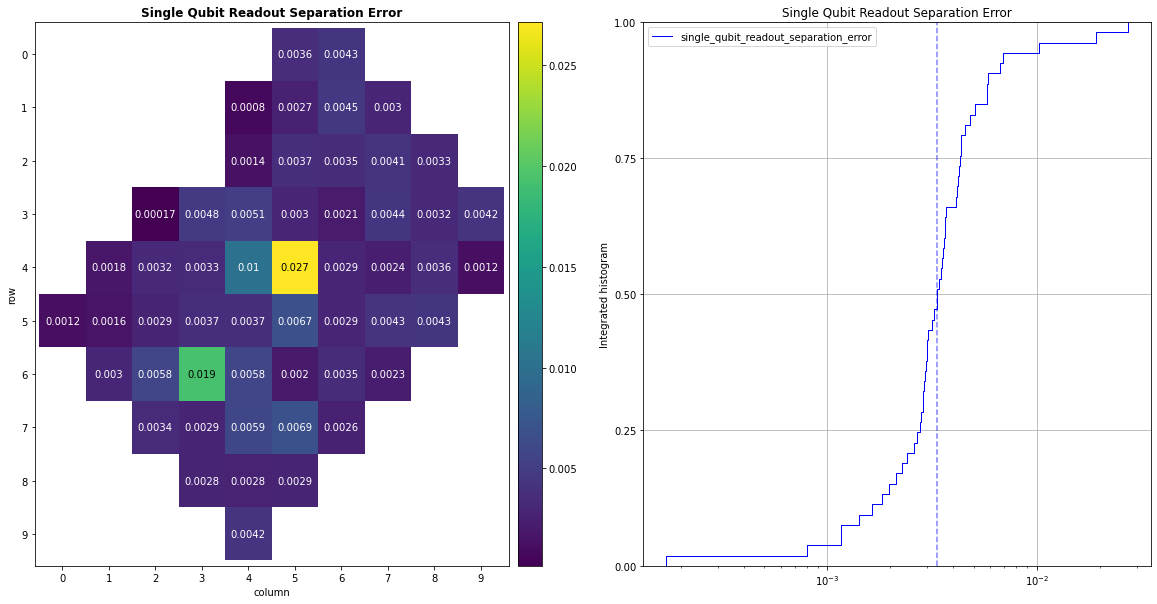The parallel single qubit readout error is when the readout for all qubits is measured at the same time.

### Parallel P00 Error

Disclaimer: The calibration metrics shown here do not correspond to actual production metrics.

``````fig, _ = cals.plot('parallel_p00_error', fig=plt.figure(figsize=(20, 10)))
``````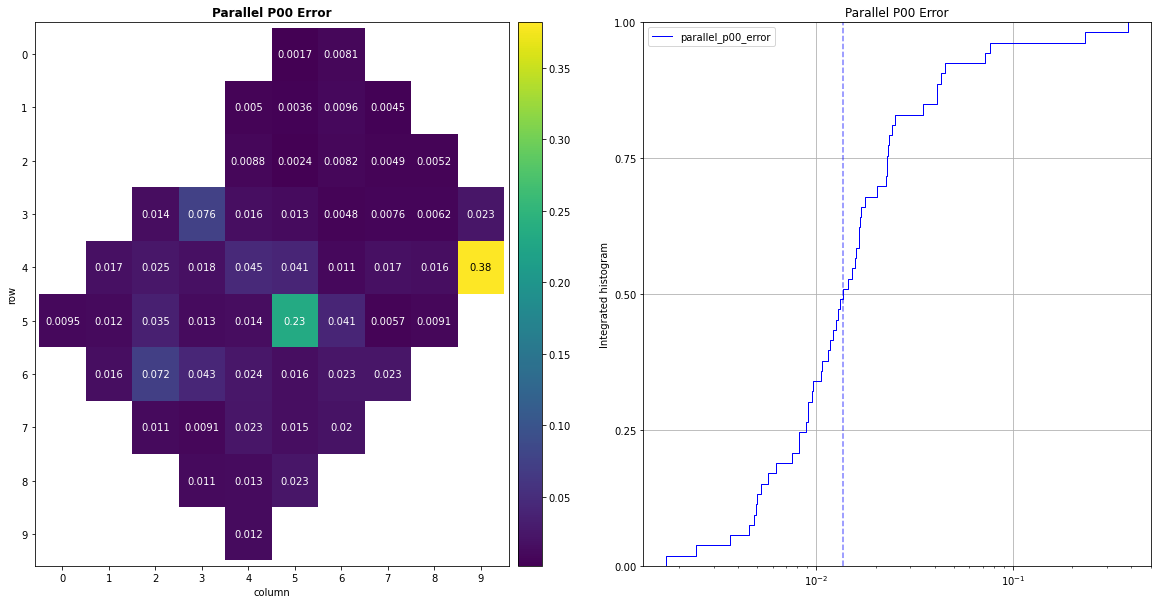### Parallel P11 Error

Disclaimer: The calibration metrics shown here do not correspond to actual production metrics.

``````fig, _ = cals.plot('parallel_p11_error', fig=plt.figure(figsize=(20, 10)))
``````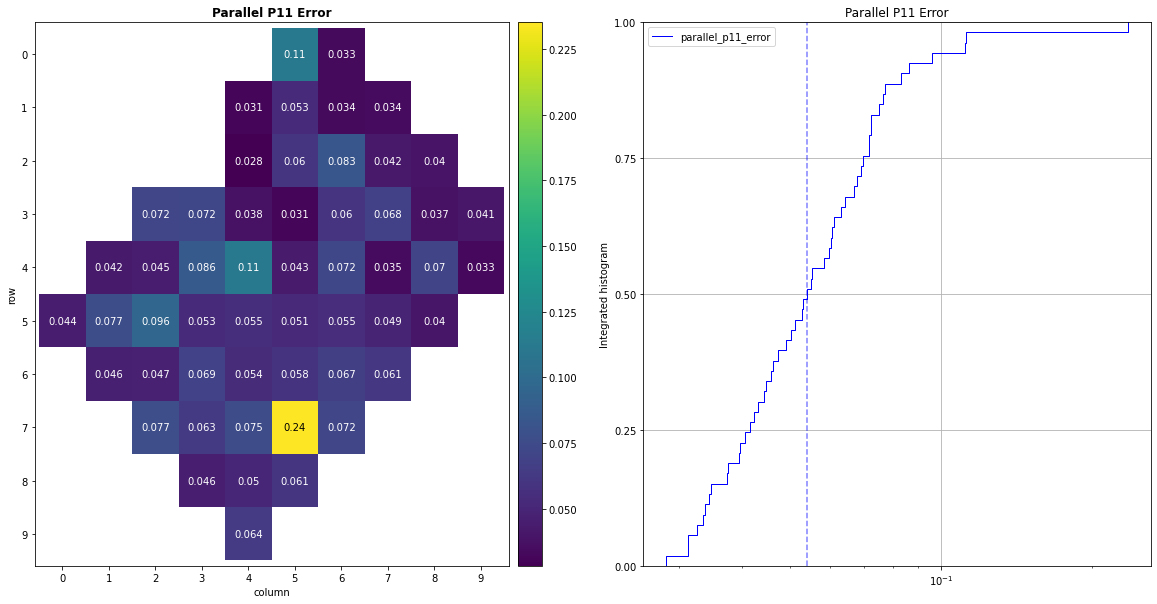## Isolated Pair XEB, sqrt(iswap)

Two qubit error is primarily characterized by applying cross-entropy benchmarking (XEB). These errors are isolated, meaning that, during the metric measurement, only the pair of qubits being considered is active. All other qubits are idle.

### Average Error

Disclaimer: The calibration metrics shown here do not correspond to actual production metrics.

``````fig, _ = cals.plot('two_qubit_sqrt_iswap_gate_xeb_average_error_per_cycle', fig=plt.figure(figsize=(20, 10)))
``````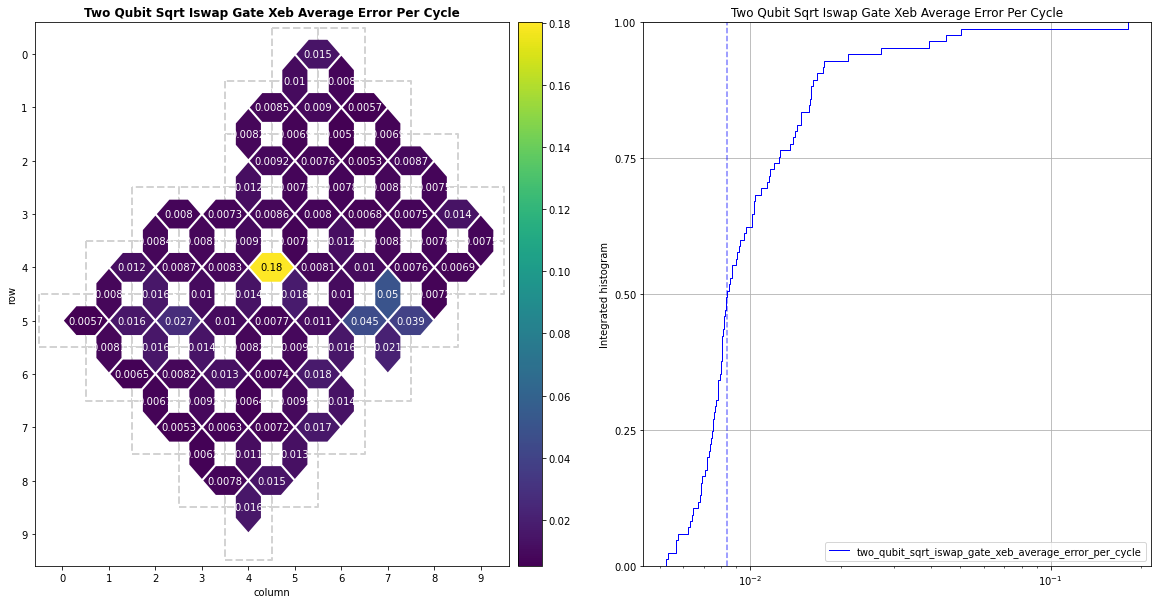### Incoherent Error

Disclaimer: The calibration metrics shown here do not correspond to actual production metrics.

``````fig, _ = cals.plot('two_qubit_sqrt_iswap_gate_xeb_incoherent_error_per_cycle', fig=plt.figure(figsize=(20, 10)))
``````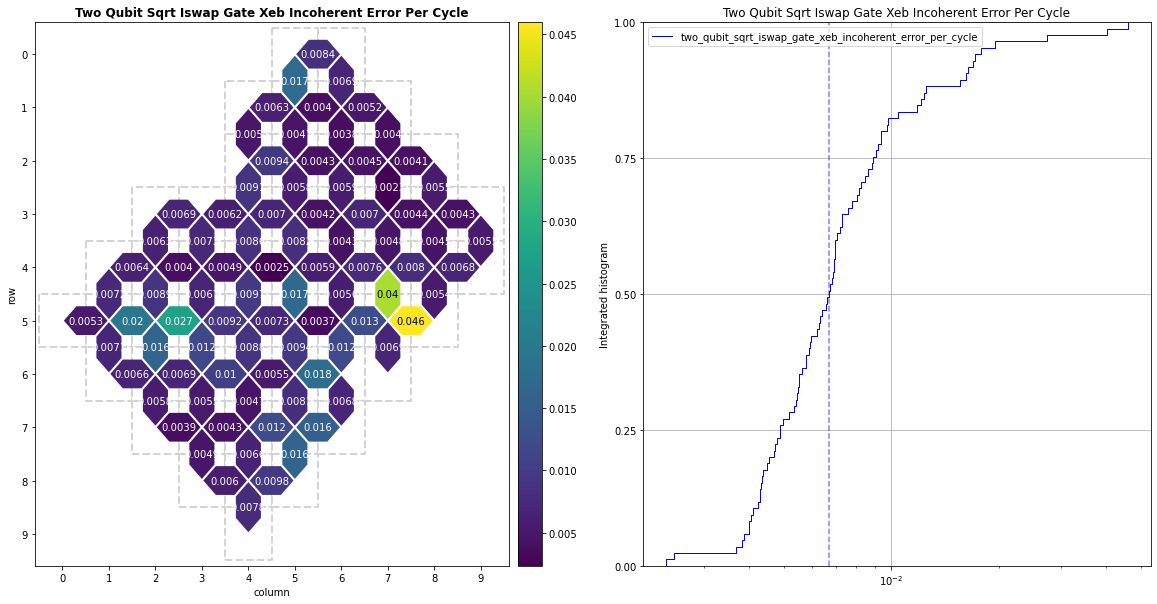### Pauli Error

Disclaimer: The calibration metrics shown here do not correspond to actual production metrics.

``````fig, _ = cals.plot('two_qubit_sqrt_iswap_gate_xeb_pauli_error_per_cycle', fig=plt.figure(figsize=(20, 10)))
``````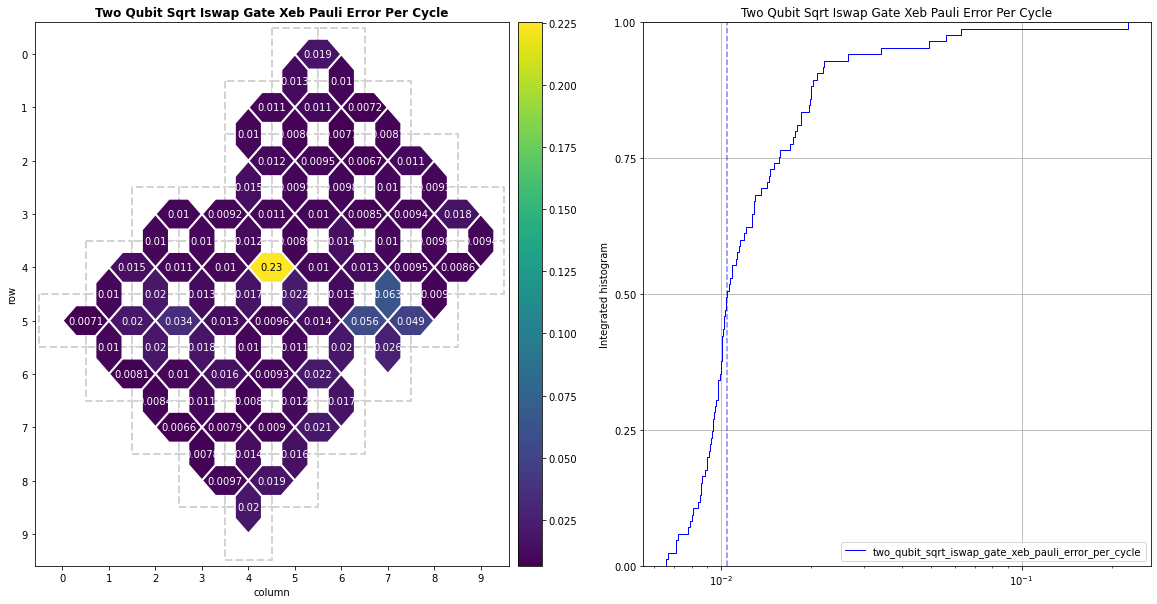## Parallel Pair XEB, sqrt(iswap)

These metrics are calculated the same way as the 2-qubit isolated XEB error metrics. However, this metric quantifies the error of multiple parallel 2-qubit cycles at a time.

### Average Error

Disclaimer: The calibration metrics shown here do not correspond to actual production metrics.

``````fig, _ = cals.plot('two_qubit_parallel_sqrt_iswap_gate_xeb_average_error_per_cycle', fig=plt.figure(figsize=(20, 10)))
``````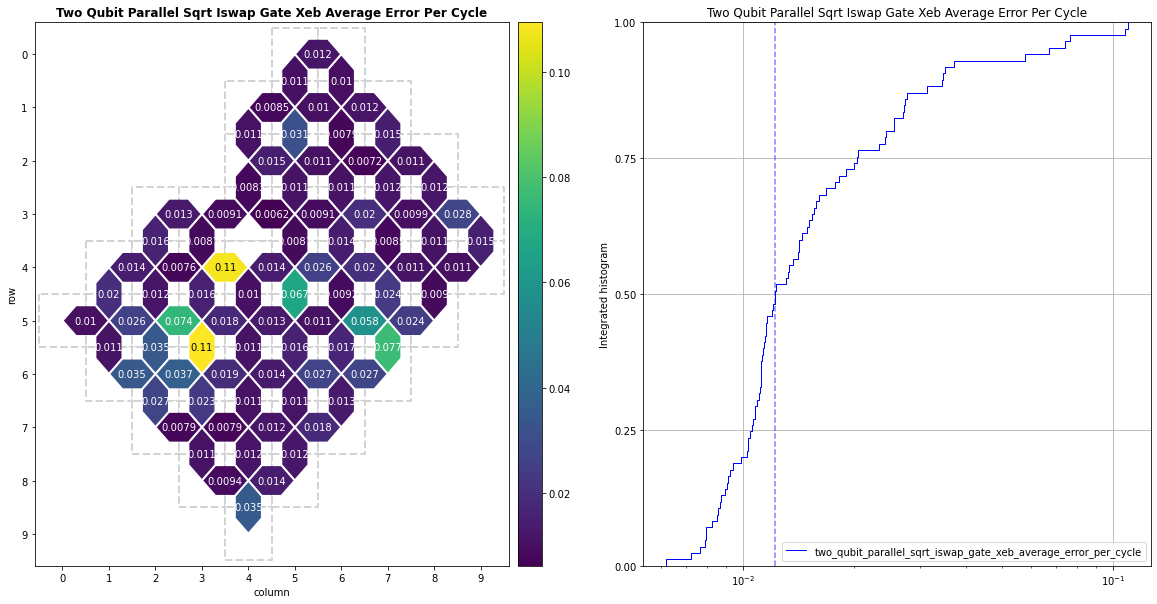### Incoherent Error

Disclaimer: The calibration metrics shown here do not correspond to actual production metrics.

``````fig, _ = cals.plot('two_qubit_parallel_sqrt_iswap_gate_xeb_incoherent_error_per_cycle', fig=plt.figure(figsize=(20, 10)))
``````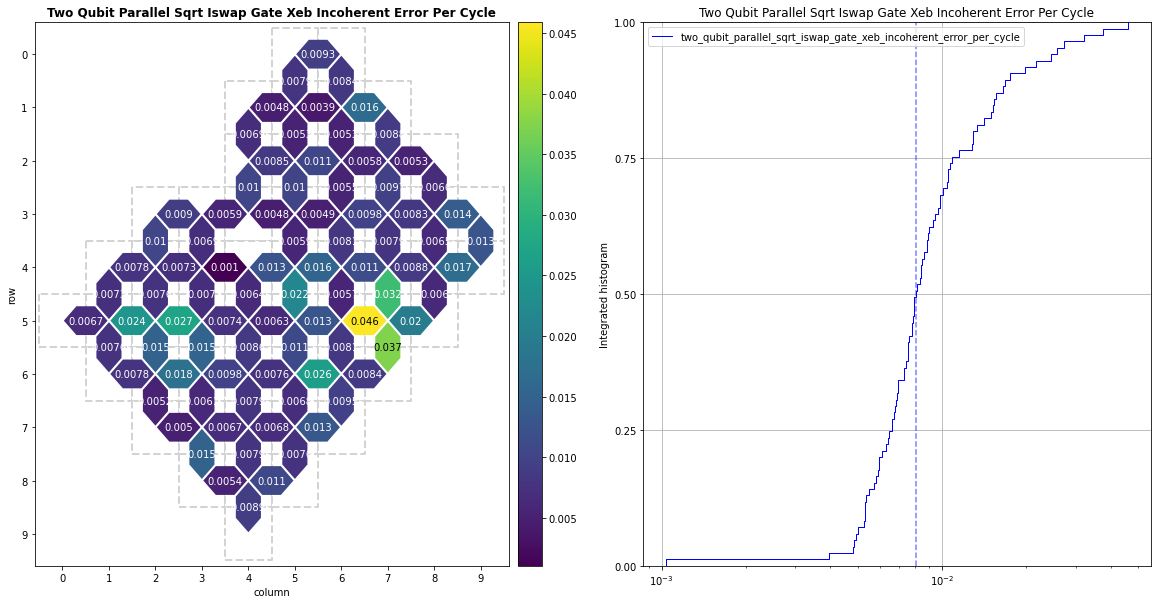### Pauli Error

Disclaimer: The calibration metrics shown here do not correspond to actual production metrics.

``````fig, _ = cals.plot('two_qubit_parallel_sqrt_iswap_gate_xeb_pauli_error_per_cycle', fig=plt.figure(figsize=(20, 10)))
``````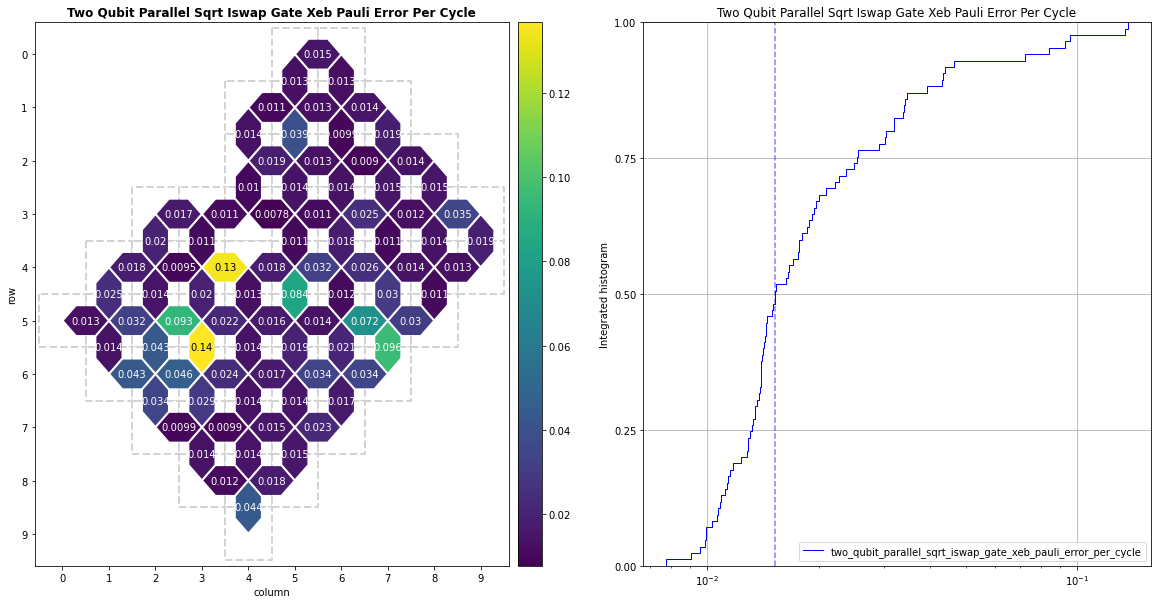[{ "type": "thumb-down", "id": "missingTheInformationINeed", "label":"Missing the information I need" },{ "type": "thumb-down", "id": "tooComplicatedTooManySteps", "label":"Too complicated / too many steps" },{ "type": "thumb-down", "id": "outOfDate", "label":"Out of date" },{ "type": "thumb-down", "id": "samplesCodeIssue", "label":"Samples / code issue" },{ "type": "thumb-down", "id": "otherDown", "label":"Other" }]
[{ "type": "thumb-up", "id": "easyToUnderstand", "label":"Easy to understand" },{ "type": "thumb-up", "id": "solvedMyProblem", "label":"Solved my problem" },{ "type": "thumb-up", "id": "otherUp", "label":"Other" }]